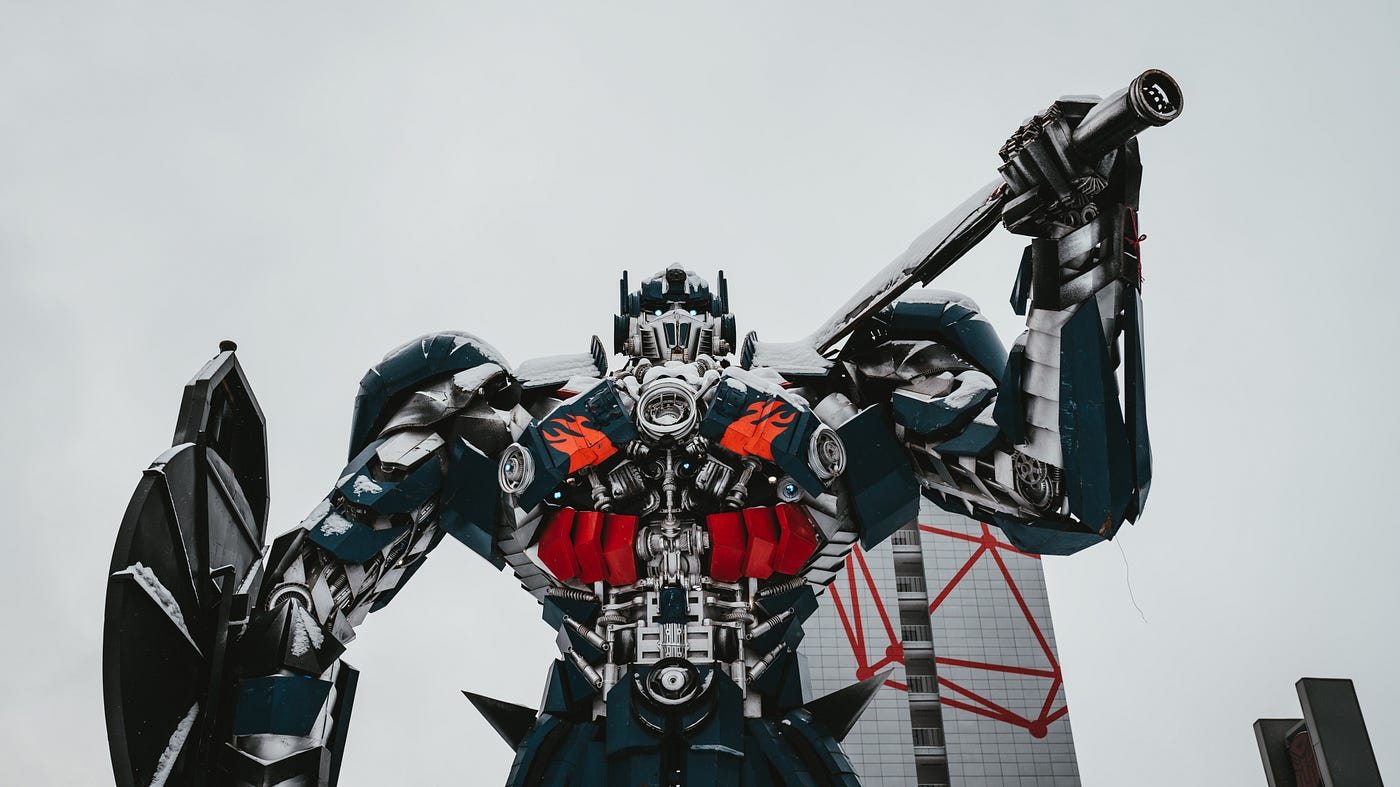# The Quantum Transformer

This is the architecture…Photo by Arseny Togulev on Unsplash

The Quantum Transformer looks like this (this is its architecture):

This is the architecture for a zero:

And, a one:

This means we can re-engineer everything in technology (everything in physics) (everything in mathematics) by understanding, fully, the conservation of a circle.

That is, any movement, from zero to one (from circumference to diameter), articulates the opposing movement, automatically (from one to zero) (from diameter to circumference).

This means there is an infinite amount of redundancy in all human systems.

Before you move forward in engineering, you have to understand what’s missing in basic mathematics: the diagram for representation (the diagram above) (representation as a noun) (representation as a verb).

Everything in Nature, and, therefore, everything in ‘reality,’ is based on the circular relationship between a noun and a verb. Meaning it is impossible to have one without the other.

This is what’s missing in quantum mechanics (our current understanding of ‘quantum’ mechanics).

Therefore, we will have to live with the massive redundancy in human systems until we have fully embraced (and ‘figured’ out) the diagram above.

Meaning absolute zero is perpetual motion giving us a current and a field (electricity and magnetics) (electromagnetics). Also, semi-conductors.

Where all of these are dependent on the zero (circumference) and the one (diameter).

Meaning, it’s unnecessary to figure out what ‘language’ is ‘saying.’ It’s always, and, only, saying, what’s exactly in the diagram. Meaning any word (any grammatical element) and-or any number (any symbol whatsoever) articulates the diagram (and the diagram articulates the word, number, symbol.)

We have to ask ourselves, before we do anything else, why do we need, or want, a quantum transformer?

It’s because we need to conserve a circle (many circles) (an infinite number of circles) because that’s what Nature dictates.

Conservation of the Circle produces a universal architecture for, because it is the core, and, thus, the only, and thus, the most important, dynamic, in Nature.